# The Azimuth Project Blog - statistical laws of Darwinian evolution

This page is a blog article in progress, written by Matteo Smerlak. To see a discussion of this article while it was being written, visit the Azimuth Forum.

guest post by Matteo Smerlak

Biologists like Steven J. Gould like to emphasize that evolution is unpredictable. They have a point: there is absolutely no way an alien visiting the Earth 400 millions years ago could have said

Hey, I know what’s gonna happen here. Some descendants of those ugly fish will grow wings and start flying in the air. Others will walk the surface of the Earth for a few million years, but they’ll get bored and they’ll eventually go back to the oceans; when they do, they’ll be able to chat across thousands of kilometers using ultrasounds. Yet others will grow arms, legs, fur, they’ll climb trees and invent BBQ, and, sooner or later, they’ll start wondering “why all this?”.

Nor can we tell if, a week from now, the flu virus will mutate, become highly pathogenic and forever remove the furry creatures from the surface of the Earth.

Evolution isn’t gravity—we can’t tell in which directions things will fall down.

One reason we can’t predict the outcomes of evolution is that genomes evolve in a super-high dimensional combinatorial space, which a ginormous number of possible turns at every step. Another is that living organisms interact with one another in a massively non-linear way, with, feedback loops, tipping points and all that jazz.

Life’s a mess, if you want my physicist’s opinion.

But that doesn’t mean that nothing can be predicted. Think of statistics. Nobody can predict who I’ll vote for in the next election, but it’s easy to tell what the distribution of votes in the country will be like. Thus, for continuous variables which arise as sums of large numbers of independent components, the central limit theorem tells us that the distribution will always be approximately normal. Or take extreme events: the max of $N$ independent random variables is distributed according to a member of a one-parameter family of so-called “extreme value distributions”: this is the content of the famous Fisher–Tippett–Gnedenko theorem.

So this is the problem I want to think about in this blog post: is evolution ruled by statistical laws? Or, in physics terms: does it exhibit some form of universality?

### Fitness distributions are the thing

One lesson from statistical physics is that, to uncover universality, you need to focus on relevant variables. In the case of evolution, it was Darwin’s main contribution to figure out the main relevant variable: the average number of viable offspring, aka fitness, of an organism. Other features—physical strength, metabolic efficiency, you name it—matter only insofar as they are correlated with fitness. If we further assume that fitness is (approximately) heritable, meaning that descendants have the same fitness as their ancestors, we get a simple yet powerful dynamical principle called natural selection: in a given population, the lineage with the highest fitness eventually dominates, i.e. its fraction goes to one over time. This principle is very general: it applies to genes and species, but also to non-living entities such as algorithms, firms or language. The general relevance of natural selection as a evolutionary force is sometimes referred to as “Universal Darwinism”.

The general idea of natural selection is pictured below (reproduced from this paper):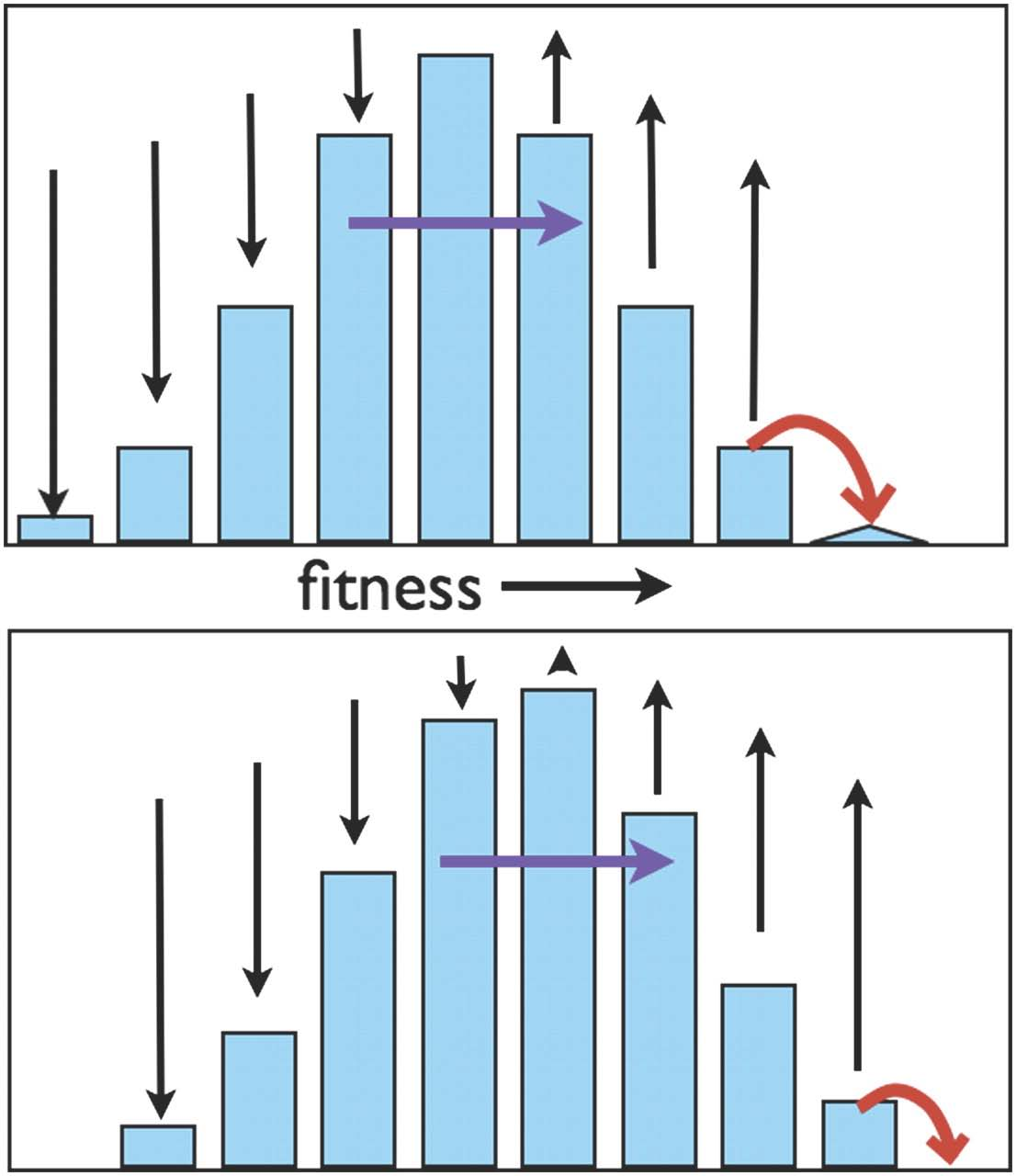It’s not hard to write down an equation which expresses natural selection in general terms. Consider an infinite population in which each lineage grows with some rate $x$. (This rate is called the log-fitness or Malthusian fitness to contrast it with the number of viable offspring $x=e^{x\Delta t}$ with $\Delta t$ the lifetime of a generation. It’s more convenient to use $x$ than $w$ in what follows, so we’ll just call $x$ “fitness”). Then the distribution of fitness at time $t$ satisfies the equation

$\displaystyle{ \frac{\partial p_t(x)}{\partial t} =\left(x-\int d y\, y\, p_t(y)\right)p_t(x) }$

whose explicit solution in terms of the initial fitness distribution $p_0(x):$

$\displaystyle{ p_t(x)=\frac{e^{x t}p_0(x)}{\int d y\, e^{y t}p_0(y)} }$

is called the Cramér transform of $p_0(x)$ in large deviations theory. That is, viewed as a flow in the space of probability distributions, natural selection is nothing but a time-dependent exponential tilt. (These equations and the results below can be generalized to include the effect of mutations, which are critical to maintain variation in the population, but we’ll skip this here to focus on pure natural selection. See my paper referenced below for more information.)

An immediate consequence of these equations is that the mean fitness $\mu_t=\int dx\, x\, p_t(x)$ grows monotonically in time, with a rate of growth given by the variance $\sigma_t^2=\mu_t=\int dx\, (x-\mu_t)^2\, p_t(x)$:

$\displaystyle{ \frac{d\mu_t}{dt}=\sigma_t^2\geq 0 }$

The great geneticist Ronald Fisher (yes, the one in the extreme value theorem!) was very impressed with this relationship. He thought it amounted to an biological version of the second law of thermodynamics, writing in his 1930 monograph

Professor Eddington has recently remarked that “The law that entropy always increases—the second law of thermodynamics—holds, I think, the supreme position among the laws of nature”. It is not a little instructive that so similar a law should hold the supreme position among the biological sciences.

Unfortunately, this excitement hasn’t been shared by the biological community, notably because this Fisher “fundamental theorem of natural selection” isn’t predictive: the mean fitness $\mu_t$ grows according to the fitness variance $\sigma_t^2$, but what determines the evolution of $\sigma_t^2$? I can’t use the identity above to predict the speed of evolution in any sense. Geneticists say it’s “dynamically insufficient”.

### Two limit theorems

But the situation isn’t as bad as it looks. The evolution of $p_t(x)$ may be decomposed into the evolution of its mean $\mu_t$, of its variance $\sigma_t^2$, and of its shape or type $\overline{p}_t(x)=\sigma_t p_t(\sigma_t x+\mu_t)$. (We also call $\overline{p}_t(x)$ the “standardized fitness distribution”.) With Ahmed Youssef we showed that:

• If $p_0(x)$ is supported on the whole real line and decays at infinity as

$-\ln\int_x^{\infty}p_0(y)d y\underset{x\to\infty}{\sim} x^{\alpha},$

for some $\alpha > 1$, then $\mu_t\sim t^{\overline{\alpha}-1}$, $\sigma_t^2\sim t^{\overline{\alpha}-2}$ and $\overline{p}_t(x)$ converges to the standard normal distribution as $t\to\infty$. Here $\overline{\alpha}$ is the conjugate exponent to $\alpha$, i.e. $1/\overline{\alpha}+1/\alpha=1$.

• If $p_0(x)$ has a finite right-end point $x_+$ with

$p(x)\underset{x\to x_+}{\sim} (x_+-x)^\beta,$

for some $\beta\geq0$, then $x_+-\mu_t\sim t^{-1}$, $\sigma_t^2\sim t^{-2}$ and $\overline{p}_t(x)$ converges to the flipped gamma distribution

$\displaystyle{ p^*_\beta(x)=\frac{(1+\beta)^{(1+\beta)/2}}{\Gamma(1+\beta)}\Theta[x-(1+\beta)^{1/2}]\,e^{-(1+\beta)^{1/2}[(1+\beta)^{1/2}-x]}\Big[(1+\beta)^{1/2}-x\Big]^\beta }$

Here and below the symbol $\sim$ means “asymptotically equivalent up to a positive multiplicative constant”; $\Theta(x)$ is the Heaviside step function. Note that $p^*_\beta(x)$ becomes Gaussian in the limit $\beta\to\infty$, i.e. the attractors of cases 1 and 2 form a continuous line in the space of probability distributions; the other extreme case, $\beta\to0$, corresponds to a flipped exponential distribution.

The one-parameter family of attractors $p_\beta^*(x)$ is plotted below: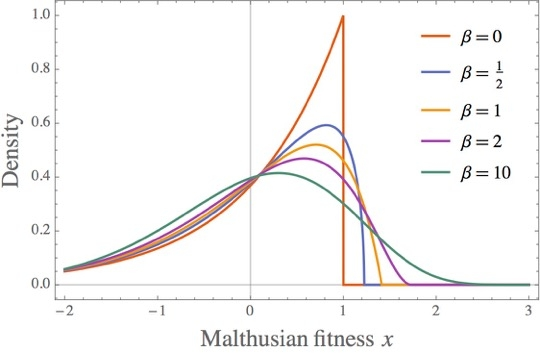These results achieve two things. First, they resolve the dynamical insufficiency of Fisher’s fundamental theorem by giving estimates of the speed of evolution in terms of the tail behavior of the initial fitness distribution. Second, they show that natural selection is indeed subject to a form of universality, whereby the relevant statistical structure turns out to be finite dimensional, with only a handful of “conserved quantities” (the $\alpha$ and $\beta$ exponents) controlling the late-time behavior of natural selection. This amounts to a large reduction in complexity and, concomitantly, an enhancement of predictive power.

(For the mathematically-oriented reader, the proof of the theorems above involves two steps: first, translate the selection equation into a equation for (cumulant) generating functions; second, use a suitable Tauberian theorem—the Kasahara theorem—to relate the behavior of generating functions at large values of their arguments to the tail behavior of $p_0(x)$. Details in our paper.)

It’s useful to consider the convergence of fitness distributions to the attractors $p_\beta^*(x)$ for $0\leq\beta\leq \infty$ in the skewness-kurtosis plane, i.e. in terms of the third and fourth cumulants of $p_t(x)$.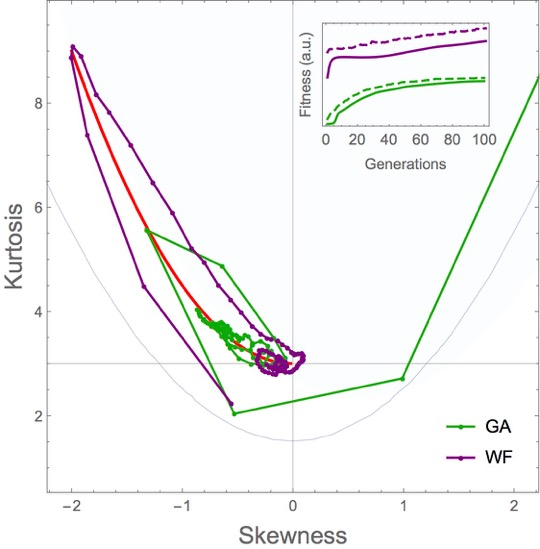The red curve is the family of attractors, with the normal at the bottom right and the flipped exponential at the top left, and the dots correspond to numerical simulations performed with the classical Wright-Fisher model and with a simple genetic algorithm solving a linear programming problem. The attractors attract!

### Conclusion and a question

Statistics is useful because limit theorems (the central limit theorem, the extreme value theorem) exist. Without them, we wouldn’t be able to make any population-level prediction. Same with statistical physics: it only because matter consists of large numbers of atoms, and limit theorems hold (the H-theorem, the second law), that macroscopic physics is possible in the first place. I believe the same perspective is useful in evolutionary dynamics: it’s true that we can’t predict how many wings birds will have in ten million years, but we can tell what shape fitness distributions should have if natural selection is true.

I’ll close with an open question for you, the reader. In the central limit theorem as well as in the second law of thermodynamics, convergence is driven by a Lyapunov function, namely entropy. (In the case of the central limit theorem, it’s a relatively recent result by Arstein et al.: the entropy of the normalized sum of $n$ i.i.d. random variables, when it’s finite, is a monotonically increasing function of $n$.) In the case of natural selection for unbounded fitness, it’s clear that entropy will also be eventually monotonically increasing—the normal is the distribution with largest entropy at fixed variance and mean.

Yet it turns out that, in our case, entropy isn’t monotonic at all times; in fact, the closer the initial distribution $p_0(x)$ from the normal, the later the entropy of the standardized fitness distribution starts to increase. Or, equivalently, the closer the initial distribution $p_0(x)$ from the normal, the later its relative entropy with respect to the normal. Why is this? And what’s the actual Lyapunov function for this process (i.e., what functional of the standardized fitness distribution is monotonic at all times under natural selection)?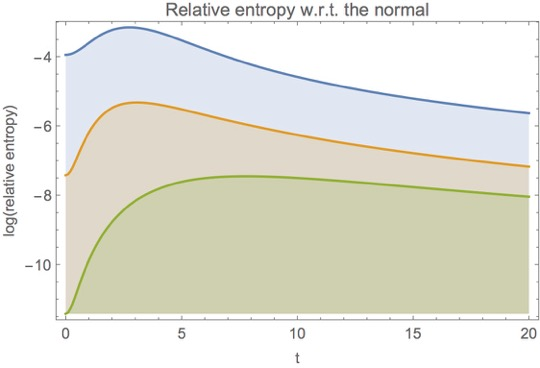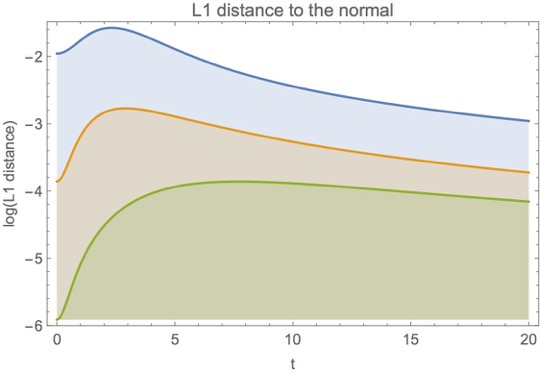In the plots above the blue, orange and green lines correspond respectively to

$\displaystyle{ p_0(x)\propto e^{-x^2/2-x^4}, \quad p_0(x)\propto e^{-x^2/2-.01x^4}, \quad p_0(x)\propto e^{-x^2/2-.001x^4} }$

### References

• S. J. Gould, Wonderful Life: The Burgess Shale and the Nature of History, W. W. Norton & Co., New York, 1989.

• M. Smerlak and A. Youssef, Limiting fitness distributions in evolutionary dynamics, 2015.

• R. A. Fisher, The Genetical Theory of Natural Selection, Oxford University Press, Oxford, 1930.

• S. Artstein, K. Ball, F. Barthe and A. Naor, Solution of Shannon’s problem on the monotonicity of entropy, J. Am. Math. Soc. 17 (2004), 975–982.

category: blog